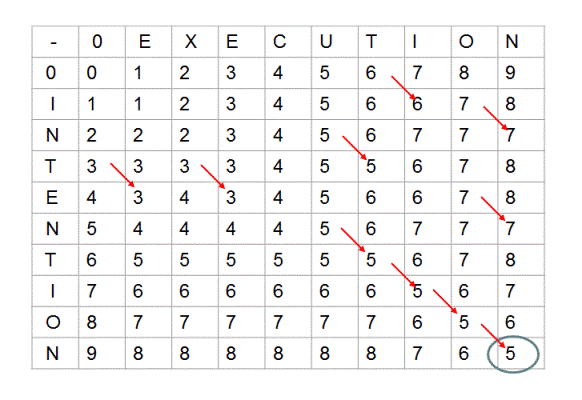# [算法笔记2]动态规划• 乌龟棋
• $pos \leftarrow i+j \times 2+k \times 3+l \times 4$
• $dp[i+1][j][k][l]=dp[i][j][k][l]+s[pos+1]$
• $dp[i][j+1][k][l]=dp[i][j][k][l]+s[pos+2]…$ 以此类推
• Codeforces 1114D. Flood Fill
• $dp[l-1][r]=\min \{ dp[l][r] + diff(a[l - 1], a[l]), dp[l][r] + diff(a[l - 1], a[r]) \}$
• $dp[l][r+1]=\min \{ dp[l][r] + diff(a[r + 1], a[r]), dp[l][r] + diff(a[r + 1], a[l]) \}$
• Codeforces 1132F. Clear the String
• $dp[l][r] = \max \{1 + dp[l + 1][r], \min \limits_{l < k \le r, s_k = s_r} dp[l + 1][k - 1] + dp[k][r] \}$

[算法笔记2]动态规划

https://kaitohh.com/oj-2/

KaitoHH

2019-03-11

2019-03-11